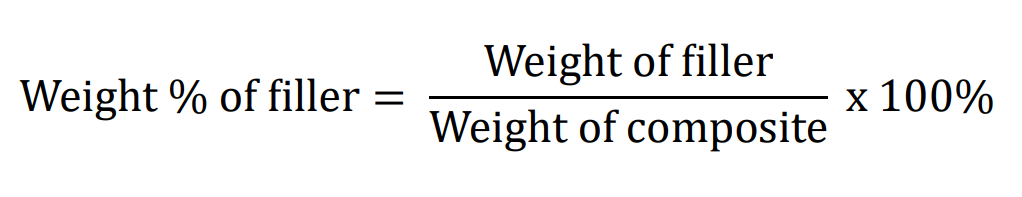# How to calculate the weight percentage of the additive?

## To calculate the weight percentage, the weight of the additive must be divided by the weight of composite and multiplied by 100%.

The weight percentage of additives in a polymer composite matrix quantifies the proportion of additive material within the overall composite. The weight percentage of additives provides insights into the composite's composition. A higher percentage indicates a greater proportion of additive, which can lead to improved specific properties. However, an excessive additive content might compromise the matrix's integrity. Proper calculation ensures that the desired properties are achieved for specific applications.

How can I calculate the weight percentage of filler?
To calculate the weight percentage of filler in a polymer composite matrix, the following formula can be
applied:The weight of the additive is the mass of the additive material added to the polymer matrix and weight of composite if the total mass of the material (polymer matrix + additive)